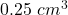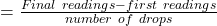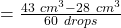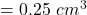## 60 drops fell from a burret.The first and final readings were 28cm³ and 43cm³ respectively.what is the average volume of one drop​

Question

60 drops fell from a burret.The first and final readings were 28cm³ and 43cm³ respectively.what is the average volume of one drop​

in progress 0
5 months 2021-08-24T23:31:29+00:00 2 Answers 1 views 0Explanation:

Data provided in the question

Number of drops fell from a burret = 60

The first reading is 28 cm^3

And, the final reading is 43 cm^3

Based on the above information, the average volume of one drop isBasically we applies the above formula by applying the three items given in the question so that the average volume of one drop could come

0.25\ cm^30.25 cm

3

Explanation:

Data provided in the question

Number of drops fell from a burret = 60

The first reading is 28 cm^3

And, the final reading is 43 cm^3

Based on the above information, the average volume of one drop is

number of drops

= \frac{43\ cm^3 – 28\ cm^3}{60 \ drops}=

60 drops

43 cm

3

−28 cm

3

= 0.25\ cm^3=0.25 cm

3